# Pupils

There are 32 pupils in the classroom, and girls are two-thirds more than boys. a) How many percents are more girls than boys? Round the result to a whole percentage. b) How many boys are in the class? c) Find the ratio of boys and girls in the class. Write in the simplest form.

c =  12
d =  20
p =  67 %
f =  3:5

### Step-by-step explanation:

c+d=32
d = c+ 2/3c

c+d=32
d = c+ 2/3•c

c+d = 32
5c-3d = 0

c = 12
d = 20

Our linear equations calculator calculates it.
$f=\frac{c}{d}=\frac{12}{20}=\frac{3}{5}=0.6=3:5$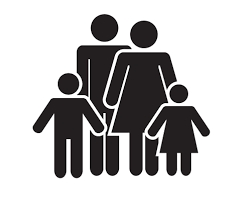Did you find an error or inaccuracy? Feel free to write us. Thank you!Tips to related online calculators
Need help to calculate sum, simplify or multiply fractions? Try our fraction calculator.
Check out our ratio calculator.
Do you have a system of equations and looking for calculator system of linear equations?
Do you want to round the number?

## Related math problems and questions:

• In the classroomThere are 30 boys and a few girls in the class. In the six months, 28 boys and all girls benefited, which was 95% of all pupils. How many pupils are there in the classroom?
• Boys and girls 8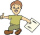In a class of 35 boys and girls that has a ratio of 2:5, 5 boys are absent and 5 boys are present. How many students are present?
• Classroom 4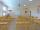In a class of 36 pupils, 2/3 are girls. How much it is in a class girls and boys?
• Prospering studentsThere are girls and 30 boys in the class. Boys prospering 28, girls all. How many girls are in the class and how many boys are there, if all the prospering students are 95%?
• ClassroomThere are eighty more girls in the class than boys. Boys are 40 percent and girls are 60 percent. How many are boys and how many girls?
• Glasses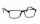There are 36 pupils in the class. Nine girls wear glasses. Boys with glasses are five less than girls without glasses. Boys without glasses are two times more than girls without glasses. How many boys and how many girls?
• Boys to girlsThe ratio of boys to girls at a party is 3:5. If six more boys arrived and four girls left the party, the ratio of boys to girls would be 5:6. How many people were at the party initially?
• Classroom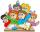In a class are 32 pupils. Boys are 8 less than girls. How many boys and girls are in the classroom?
• ClassIn a class are 32 pupils. Of these are 8 boys. What percentage of girls are in the class?
• ClassroomOf the 26 pupils in the classroom, 12 boys and 14 girls, four representatives are picked to the odds of being: a) all the girls b) three girls and one boy c) there will be at least two boys
• GirlsThe boys and girls in the class formed groups without the rest of the fives, two girls and three boys. There are six girls missing to create mixed pairs (1 boy and one girl). How many girls are in the classroom?
• Classroom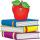In a classroom are 30 students, boys are four times more than girls. How many boys are in the class?
• PupilsThere are 350 girls in the school, and the other 30% of the total number of pupils are boys. How many pupils does the school have?
• Ski class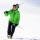Class attend 30 boys and some number of girls. Ski training was attended by 28 boys and all the girls, which was 95% of all students. How many girls attend this class? How many percent is that?
• Cross-country competition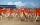Cross-country competition was attended by 76% of pupils of class 9.B. Class consis of 16 boys, two of whom did not run and 4 girls did not run. How many girls go to class 9.B?
• The classroom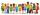In the seventh class the number of boys and girls are at a ratio of 4:5. Boys are 16. How many total students are in the classroom?
• Summer camp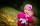84 children spent their holidays at the summer camp. There were 8 fewer boys than girls. What percentage were boys and what percentage were girls?